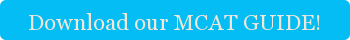The Official Cambridge Coaching Approach to MCAT Thermochemistry Questions (patent pending)

Thermochemistry is hard. You have to deal with a bunch of imaginary concepts like enthalpy, entropy, and the dreaded Gibbs free energy. These concepts are not very intuitive and are riddled with pitfalls UNLESS you have an awesome Cambridge Coaching tutor to make it CRYSTAL clear for you.

Let's start with equilibrium constants (K). These constants tell us if a reaction's equilibrium lies to the right (favoring products) or to the left (favoring reactants). Write this down an memorize it: if K>1, the reaction favors products and if K<1 the reaction favors reactants. But where does K come from???

Well, young MCATer, K is related to delta G (aka Gibbs free energy) by the following formula:

#### Delta G=-RT ln(K)

Where delta G is the Gibbs free energy, R is the universal gas constant (8.314), T is the temperature in kelvin, and K is the equilibrium constant. Notice a couple of things. When K is less than 1, ln (K) is a negative term, and, because of the negative sign out front, delta G ends up being positive. And vice versa; when K is a number greater than 1, delta G is negative. Memorize this too: positive delta G favors the reverse reaction, negative delta G favors the forward reaction.

Now, let's bring in entropy an enthalpy. Enthalpy is a measure of how much a given reaction heats up its surroundings. It is a byproduct of the fact that stronger chemical bonds are at a lower energy than weaker ones, so the excess energy is released as heat. A reaction that has a negative enthalpy heats up its surroundings and is said to be exothermic. A reaction that has a positive enthalpy cools off its surroundings and is said to be endothermic.

Entropy, as you've probably learned, is a measure of disorder. Dissolved things have more entropy after they dissolve, gasses have more entropy than liquids, and decomposed elements, all things being equal, have more entropy than the molecules they were decomposed out of.

#### Delta G=Delta H - T *delta S

The above reaction is how Gibbs free energy relates to enthalpy (abbreviated as H) and entropy (abbreviated as S). T is temperature in Kelvins. Remember that reactions with a NEGATIVE Gibbs free energy go forward. So that means that the universe likes reactions that have negative enthalpy an positive entropy.

Does this mean that a reaction with a positive enthalpy won't go forward! Heck no! As long as entropy is high enough the delta G will still be negative. Same thing for reactions with decreasing entropy; they will still go forward so long as enthalpy is negative enough!

Here is my MCAT test tip to you: the best way to prep for thermochemistry on the MCAT is not just to memorize the formulas above (although you should memorize them). The best MCAT strategy is to develop an intuitive understanding of how these concepts relate to one another that can help you crush through MCAT problems. An MCAT tutor is a great way to help get to that point of intuitive understanding, and will make your MCAT preparation that much easier.Tags: chemistry, MCAT